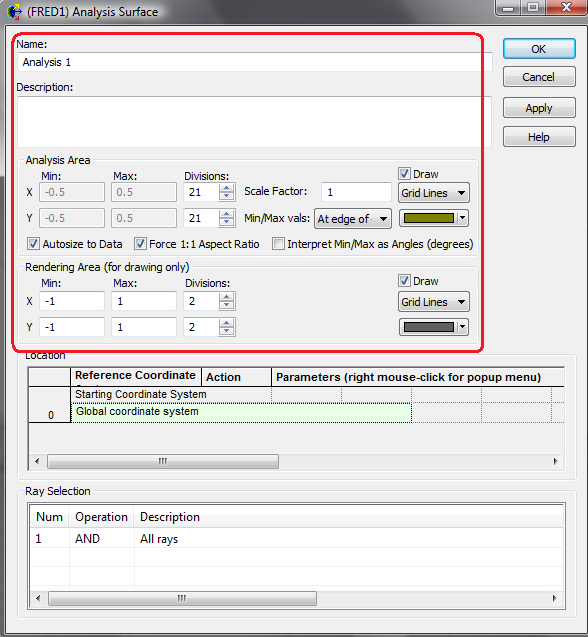# Analysis Surface Script Commands

Analysis surfaces themselves have three defining parameters, their sampling grid, ray filters, and location in space. They can also be used to generate Analysis Results Nodes(ARNs) which make viewing and exporting the data from an analysis calculation much easier. Many of the scripting commands however, also include a T_ANALYSIS data, which only defines a sampling grid and location (no ray filters).

In the scripting language, analysis surfaces entities are either defined by a T_ANALYSISSURF data structure or referenced directly by their node number. The data structure contains all of the information that describes the sampling and rendering information of the analysis surface in the FRED document, but does not contain any of the ray filter information or global position information. It essentially contains the top half of the information that is in the analysis surface dialog.The T_ANALYSIS data structure is used to define a sampling grid and the vector direction for each axis of the sampling grid, but does not contain any information about drawing parameters or ray filters. The T_ANALYSIS structure is only used for older scripting commands such as Irradiance and Intensity.

In order to create a T_ANALYSIS structure with the location and sampling information from a specific surface node in the FRED document, use the command below (information from Help). REMEMBER, this simply extracts the sampling grid from the analysis surface node.

n As Long
Node number of the entity to load the analysis structure from
ana As T_ANALYSIS
Structure loaded with the analysis data from the FRED entity (in global coordinates)

The key point to note in the scripting command is that the data loaded into the T_ANALYSIS structure is in global coordinates.

Some of the analyses commands that use the T_ANALYSIS structure have an input option to “Include in the calculation only those rays on the entity with the given node number n”. This type of argument serves as a ray filter which is then used in conjunction with the sampling grid defined by T_ANALYSIS. An example is shown below for the case of the Irradiance command.

count (Long)
The number of rays included in the calculation.
n As Long
Include in the calculation only those rays on the entity with the given node number n.
nCoordSys As Long
Node number of the FRED entity whose coordinate system the ana structure is expressed in. If the LoadAnalysis command was used to populate the T_ANALYSIS structure, the value of nCoordSys should be -1.
ana As T_ANALYSIS
Structure defining a sample area over which the calculation will be performed.
Array to be populated with results of the calculation.

In the Irradiance command shown above, the T_ANALYSIS structure provides the sampling grid in space and “n” provides the ray selection criteria. To include all rays, the value of “n” will need to be -1. If only rays on a specific surface are intended to be included in the result, then the value of n will be the node number of that surface.

As is the case with almost all calculations that can be performed in FRED, there are a set of commands that can perform the same calculation with different inputs and different methods of data output. For the Irradiance calculation, the family of commands is Irradiance, IrradianceToFile, IrradianceToFileAS, and IrradianceToARN.

In the irradiance commands referenced above, the only difference between Irradiance and IrradianceToFile is that the array storing the data in Irradiance is replaced with a file path where the data is written.

The commands that are most equivalent to using the GUI are the IrradianceToFileAS and IrradianceToARN commands, since they directly use analysis surfaces to perform the calculations. The IrradianceToARN command is outlined below.

numRays = IrradianceToARN( anaNode, arnName, arnNode )
numRays (Long)
Number of rays included in the calculation
anaNode As Long
Node number of the analysis surface used in the calculation. The analysis surface's ray filters are applied.
arnName As String
Name of the ARN being created.
arnNode As Long
Node number of the newly created ARN. Returned by the function.

The IrradianceToARN command is straightforward to use and saves the data as an ARN rather than as a temporary data array within the script. The ray filters of the analysis surface are also included in the calculation so there is no need to filter the rays manually by other scripting methods. Furthermore, there are a whole family of “ARN…” script commands that facilitate rapid manipulation, storage and display of calculations stored in analysis results nodes.

In summary, there is often some confusion as to which is the proper command to use to perform a calculation in a script. Commands which make use of T_ANALYSIS or T_ANALYSISSURF are generally older script commands and are not recommended when equivalent commands of the type IrradianceToARN (or variations) are available.

We always recommend routing the calculation through an ARN when possible since that makes available the entire family of ARN scripting commands for data manipulation and display.